## How to Calculate and Solve for Standard Normal Variable | Probability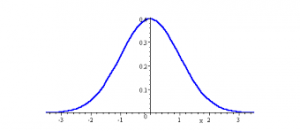The image above represents standard normal variable.

To compute for standard normal variable, three essential parameters are needed and these parameters are value (x), mean (μ) and standard deviation (σ).

The formula for calculating standard normal variable:

z = (x – μ)σ

Where;

z = Standard Normal Variable
x = Value
μ = Mean
σ = Standard Deviation

Let’s solve an example;
Find the standard normal variable when the value is 4, the mean is 20 and the standard deviation is 26.

This implies that;

x = Value = 4
μ = Mean = 20
σ = Standard Deviation = 26

z = (x – μ)σ
z = (4 – 20)26
z = (-16)26
z = -0.615

Therefore, the standard normal variable is -0.615.

## How to Calculate and Solve for Standard Deviation | Probability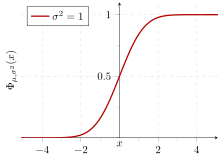The image above represents standard deviation.

To compute for standard deviation, three essential parameters are needed and these parameters are Number of possible outcomes in any single trial (n), Probability of a success in any single trial (p) and Probability of a failure in any single trial (q).

The formula for calculating standard deviation:

σ = √(npq)

Where;

σ = Standard deviation
n = Number of Possible Outcomes in Any Single Trial
p = Probability of a Success in Any Single Trial
q = Probability of a Failure in Any Single Trial

Let’s solve an example;
Find the standard deviation when the number of possible outcomes in any single trial is 14, the probability of a success in any single trial is 0 and the probability of a failure in any single trial is 1.

This implies that;

n = Number of Possible Outcomes in Any Single Trial = 14
p = Probability of a Success in Any Single Trial = 0
q = Probability of a Failure in Any Single Trial = 1

σ = √(npq)
σ = √((14)(0)(1))
σ = √(0)
σ = 0

Therefore, the standard deviation is 0.

Calculating for Number of Possible Outcomes in Any Single Trial when the Standard Deviation, the Probability of a Success in Any Single Trial and the Probability of a Failure in Any Single Trial is Given.

n = σ2 / pq

Where;

n = Number of Possible Outcomes in Any Single Trial
σ = Standard deviation
p = Probability of a Success in Any Single Trial
q = Probability of a Failure in Any Single Trial

Let’s solve an example;
Given that standard deviation is 5, the probability of a success in any single trial is 1 and the probability of a failure in any single trial is 1. Find the number of possible outcomes in any single trial?

This implies that;

σ = Standard deviation = 5
p = Probability of a Success in Any Single Trial = 1
q = Probability of a Failure in Any Single Trial = 1

n = σ2 / pq
n = 52 / 1 x 1
n = 25 / 1
n = 25

Therefore, the number of possible outcomes in any single trial is 25.

## How to Calculate and Solve for Mean | Probability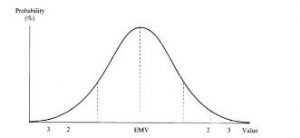The image above represents mean.

To compute for mean, two essential parameters are needed and these are number of possible outcomes in any single trial (n) and probability of success in any single trial (p).

The formula for calculating mean:

μ = np

Where;

μ = Mean
n = Number of Possible Outcomes in Any Single Trial
p = Probability of success in any single trial

Let’s solve an example;
Find the mean when the number of possible outcomes in any single trial is 8 and the probability of success in any single trial is 1.

This implies that;

n = Number of Possible Outcomes in Any Single Trial = 8
p = Probability of success in any single trial = 1

μ = np
μ = (8)(1)
μ = 8

Therefore, the mean is 8.

Calculating for the Number of Possible Outcomes in Any Single Trial when the Mean and the Probability of Success in Any Single Trial is Given.

n = μ / p

Where;

n = Number of Possible Outcomes in Any Single Trial
μ = Mean
p = Probability of Success in Any Single Trial

Let’s solve an example;
Find the number of possible outcomes in any single trial when the mean is 15 and the probability of success in any single trial is 1.

This implies that;

μ = Mean = 15
p = Probability of Success in Any Single Trial = 1

n = μ / p
n = 15 / 1
n = 15

Therefore, the number of possible outcomes in any single trial is 15.

## How to Calculate and Solve for Poisson Probability Distribution | Probability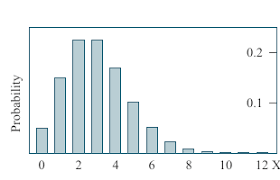The image above represents Poisson probability distribution.

To compute for Poisson probability distribution, two essential parameters are needed and these parameters are Mean of the Theoretical Distribution (μ) and Number of successes of event A (r).

The formula for Poisson probability distribution:

P(x = r) = e-μ(μr)r!

Where;

P(x = r) = Poisson Probability Distribution
r = Number of Successes of Event A
μ = Mean of the Theoretical Distribution

Let’s solve an example;
Find the poisson probability distribution when the mean of the theoretical distribution is 12 and the number of successes of event A is 6.

This implies that;

r = Number of Successes of Event A = 6
μ = Mean of the Theoretical Distribution = 12

P(x = r) = e-μ(μr)r!
P(x = r) = e-12(12)66!
P(x = r) = (0.00000614)(2985984)(720)
P(x = r) = 0.025

Therefore, the Poisson probability distribution is 0.025.

## How to Calculate and Solve for General Binomial Distribution | Probability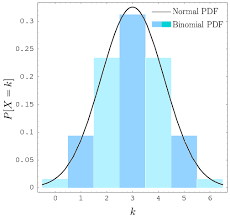The image above represents general binomial distribution.

To compute for general binomial distribution, four essential parameters are needed and these parameters are n, r, q and q.

The formula for calculating general binomial distribution:

P(r successes) = n!(n – r)!r!q(n – r)pr

Where;

P(r successes) = General Binomial Distribution
p = P(A)
q = P(not A)

Let’s solve an example;
Find the general binomial distribution when n is 8, r is 6, p is 1 and q is 0.

This implies that;

n = 8
r = 6
p = 1
q = 0

P(r successes) = n!(n – r)!r!q(n – r)pr
P(6 successes) = 8!(8 – 6)!6!(0)(8 – 6)(1)6
P(6 successes) = 8!2!6!(0)2(1)6
P(6 successes) = 40320(2)(720)(0)2(1)6
P(6 successes) = 403201440(0)2(1)6
P(6 successes) = (28)(0)2(1)6
P(6 successes) = (28)(0)(1)
P(6 successes) = 0

Therefore, the general binomial distribution is 0.

## How to Calculate and Solve for Dependent Events | Probability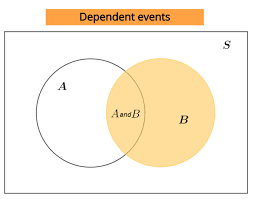The image above represents dependent events.

To compute for dependent events, four essential parameters are needed and these parameters are Number of Times Event A can occur (xA), Number of Times Event B can occur (xB) and Total Number of All Possible Outcomes (N).

The formula for calculating independent events:

P(A and B) = P(A) x P(B|A)

Where;

P(A and B) = Dependent events
xA = Number of Times Event A can occur
xB = Number of Times Event B can occur
N = Total Number of All Possible Outcomes
P(A) = xAN
P(B|A) = xB(N – 1)

Let’s solve an example;
Find the dependent events when the number of times event A can occur is 8, number of times event B can occur is 11 and the total number of all possible outcomes is 18.

This implies that;

xA = Number of Times Event A can occur = 8
xB = Number of Times Event B can occur = 11
N = Total Number of All Possible Outcomes = 18

P(A and B) = P(A) x P(B|A)
P(A and B) = xAN x xB(N – 1)
P(A and B) = 818 x 1117
P(A and B) = (8)(11)(18)(17)
P(A and B) = 88306
Dividing the numerator and denominator by 2
P(A and B) = 44153
P(A and B) = 0.287

Therefore, the dependent events is 0.287.

## How to Calculate and Solve for Independent Events | Probability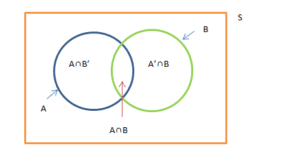The image above represents independent events.

To compute for independent events, four essential parameters are needed and these parameters are Number of Times Event A can occur (xA), Number of Times Event B can occur (xB) and Total Number of All Possible Outcomes (N).

The formula for calculating independent events:

P(A and B) = P(A) x P(B)

Where;

P(A and B) = Independent events
xA = Number of Times Event A can occur
xB = Number of Times Event B can occur
N = Total Number of All Possible Outcomes
P(A) = xAN
P(B) = xBN

Let’s solve an example;
Find the independent events when the number of times event A can occur is 11, number of times event B can occur is 15 and the total number of all possible outcomes is 22.

This implies that;

xA = Number of Times Event A can occur = 11
xB = Number of Times Event B can occur = 15
N = Total Number of All Possible Outcomes = 22

P(A and B) = P(A) x P(B)
P(A and B) = xAN x xBN
P(A and B) = 1122 x 1522
P(A and B) = (11)(15)(22)(22)
P(A and B) = 165484
Dividing the numerator and denominator by 11
P(A and B) = 1544
P(A and B) = 0.3409

Therefore, the independent events is 0.3409.

## How to Calculate and Solve for Mutually Non-Exclusive | ProbabilityThe image above represents mutually non-exclusive.

To compute for mutually non-exclusive, four essential parameters are needed and these parameters are xA, NA, xB and NB.

The formula for calculating mutually non-exclusive:

P(A or B) = P(A) + P(B) – P(A and B)

Where;

P(A or B) = Mutually Non-Exclusive
P(A) = xANA
P(B) = xBNB

Let’s solve an example;
Find the mutually non-exclusive when the xA is 10, NA is 20, xB is 5 and NB is 12.

This implies that;

xA = 10
NA = 20
xB = 5
NB = 12

P(A or B) = P(A) + P(B) – P(A and B)
P(A or B) = P(A) + P(B) – (P(A) x P(B))
P(A or B) = xANA + xBNB – (xANA x xB NB)
P(A or B) = 10 20 + 5 12 – (10 20 x 5 12)
P(A or B) = 10(12) + 5(20) (20)(12) – ((10)(5) (20)(12))
P(A or B) = 120 + 100 240 – (50 240)
P(A or B) = 220 240 – 50 240
P(A or B) = (220 – 50) 240
P(A or B) = 170 240
Dividing the numerator and denominator by 10
P(A or B) = 17 24
P(A or B) = 0.708

Therefore, the mutually non-exclusive is 0.708.

## How to Calculate and Solve for Mutually Exclusive | Probability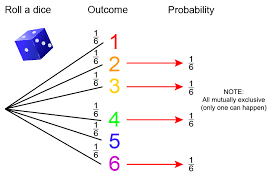The image above represents mutually exclusive.

To compute for mutually exclusive, four essential parameters are needed and these are xA, NA, xB and NB.

The formula for calculating mutually exclusive:

P(A or B) = P(A) + P(B)

Where;

P(A or B) = Mutually Exclusive
P(A) = xANA
P(B) = xBNB

Let’s solve an example;
Find the mutually exclusive when the xA is 12, NA is 14, xB is 9 and NB is 17.

This implies that;

xA = 12
NA = 14
xB = 9
NB = 17

P(A or B) = P(A) + P(B)
P(A or B) = xANA + xBNB
P(A or B) = 1214 + 917
P(A or B) = 12(17) + 9(14)(14)(17)
P(A or B) = 204 + 126238
P(A or B) = 330238
Dividing the numerator and denominator by 2
P(A or B) = 165119
P(A or B) = 1.386

Therefore, the mutually exclusive is 1.386.

## How to Calculate and Solve for Impossibility | Probability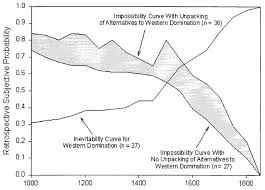The image above represents impossibility.

To compute for impossibility, one essential parameter is needed and this parameter is Total Number of All Possible Outcomes (N).

The formula for calculating impossibility:

P(A) = x N

Where;

P(A) = Imposssibility
N = Total Number of All Possible Outcomes

Let’s solve an example;
Find the impossibility when the total number of all possible outcomes is 12.

This implies that;

N = Total Number of All Possible Outcomes = 12

P(A) = x N
P(A) = 0 12
P(A) = 0

Therefore, P(impossibility) is 0.